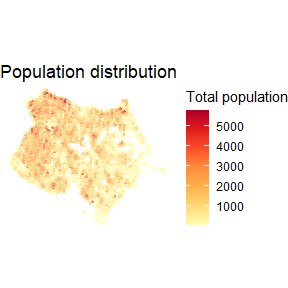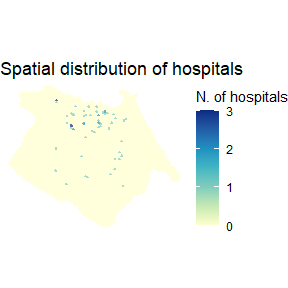# Mapping population and land use

#### 2021-04-28

Spatial distribution of population and activities

# load libraries
library(aopdata)
library(data.table)
library(ggplot2)
library(sf)

Download land use data


# download aop data
df <- read_landuse(city='Fortaleza',
year=2019,
geometry = T,
showProgress = F)

Map Population Spatial distribution population by income decile

ggplot() +
geom_sf(data=subset(df, P001>0), aes(fill=P001), color=NA, alpha=.7) +
scale_fill_distiller(palette = "YlOrRd", direction = 1)+
labs(title='Population distribution', fill="Total population") +
theme_void()Map income levels Spatial distribution population by income decile

ggplot() +
geom_sf(data=subset(df, !is.na(R002)), aes(fill=factor(R003)), color=NA, alpha=.7) +
scale_fill_brewer(palette = "RdBu") +
labs(title='Average household income per capita', fill="Income decile") +
theme_void()

Map Schools Spatial distribution of elementary schools

ggplot() +
geom_sf(data=df, aes(fill=E003), color=NA, alpha=.7) +
scale_fill_distiller(palette = "YlOrRd", direction = 1)+
labs(title='Spatial distribution of elementary schools', fill="N. of schools") +
theme_void()

Map Hospitals Spatial distribution of high-complexity health care facilities

ggplot() +
geom_sf(data=df, aes(fill=S004), color=NA, alpha=.7) +
scale_fill_distiller(palette = "YlGnBu", direction = 1)+
labs(title='Spatial distribution of hospitals', fill="N. of hospitals") +
theme_void()